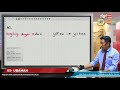#### Form 1##### MathematicsRational Numbers
Integers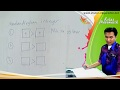Integers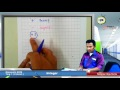Integers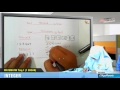Basic arithmetic operations Involving integer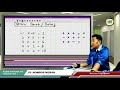Basic arithmetic operations Involving integer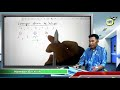Basic arithmetic operations Involving integer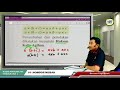Positive fractions and negative fractions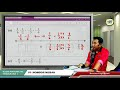Positive fractions and negative fractions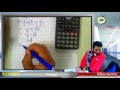Linear Inequalities
Linear inequalities in one variable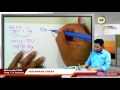Lines and Angles
Lines and angles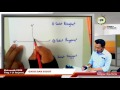Lines and angles#### Form 2##### MathematicsCoordinates
Distance in the Cartesian Coordinate System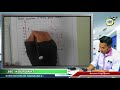#### Form 5##### MathematicsVariations
Direct Variation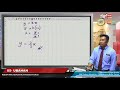Inverse Variation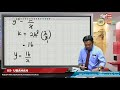Combined Variation